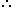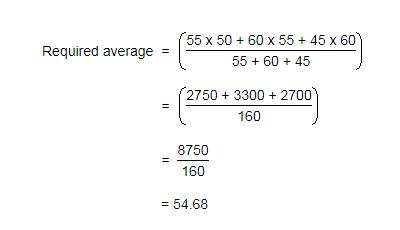Courses

# Practice Test: Averages - 2

## 10 Questions MCQ Test Quantitative Aptitude for Banking Preparation | Practice Test: Averages - 2

Description
This mock test of Practice Test: Averages - 2 for Quant helps you for every Quant entrance exam. This contains 10 Multiple Choice Questions for Quant Practice Test: Averages - 2 (mcq) to study with solutions a complete question bank. The solved questions answers in this Practice Test: Averages - 2 quiz give you a good mix of easy questions and tough questions. Quant students definitely take this Practice Test: Averages - 2 exercise for a better result in the exam. You can find other Practice Test: Averages - 2 extra questions, long questions & short questions for Quant on EduRev as well by searching above.
QUESTION: 1

### A grocer has a sale of Rs. 6435, Rs. 6927, Rs. 6855, Rs. 7230 and Rs. 6562 for 5 consecutive months. How much sale must he have in the sixth month so that he gets an average sale of Rs. 6500?

Solution:

Total sale for 5 months = Rs. (6435 + 6927 + 6855 + 7230 + 6562) = Rs. 34009.Required sale = Rs. [ (6500 x 6) - 34009 ]

= Rs. (39000 - 34009)

= Rs. 4991.

QUESTION: 2

Solution:
QUESTION: 3

### Arun obtained 76, 65, 82, 67 and 85 marks (out in 100) in English, Mathematics, Chemistry, Biology and Physics. What is his average mark?

Solution:

Average = (76 + 65 + 82 + 67 + 85 )/ 5 = 375/5 =  75.

QUESTION: 4

The average age of husband, wife and their child 3 years ago was 27 years and that of wife and the child 5 years ago was 20 years. What is the present age of the husband?

Solution:

Sum of the present ages of husband, wife and child = (27 * 3 + 3 * 3) years = 90 years.
Sum of the present ages of wife and child = (20 * 2 + 5 * 2) years = 50 years.
Husband's present age = (90 - 50) years
= 40 years

QUESTION: 5

If the average marks of three batches of 55, 60 and 45 students respectively is 50, 55, 60, what is the average marks of all the students?

Solution:QUESTION: 6

The average weight of A, B and C is 45 kg. If the average weight of A and B be 40 kg and that of B and C be 43 kg, what is the weight of B?

Solution:

Let A, B, C represent their respective weights. Then, we have:

A + B + C = (45 x 3) = 135 .... (i)

A + B = (40 x 2) = 80 .... (ii)

B + C = (43 x 2) = 86 ....(iii)

Adding (ii) and (iii), we get: A + 2B + C = 166 .... (iv)

Subtracting (i) from (iv), we get : B = 31.B's weight = 31 kg.

QUESTION: 7

The average weight of 8 person's increases by 2.5 kg when a new person comes in place of one of them weighing 65 kg. What is the weight of the new person?

Solution:

The average weight of 8 persons increases by 2.5 kg
Total weight of 8 persons increased = 8 × 2.5 kg = 20 kg.
New person comes in place of one of them weighing 65 kg.
Hence the increase of weight of 8 persons together is because of new person replacing the person having 65 kg weight. Accordingly
Weight of new person = 65 + 20 kg = 85 kg
Thus the person replacing the 65 kg person should have 85 kg weight, which causes increase of weight of the group together by 2.5 kg .

QUESTION: 8

A student needed to find the arithmetic mean of the numbers 3, 11, 7, 9, 15, 13, 8, 19, 17, 21, 14 and x. He found the mean to be 12. What is the value of x?

Solution:

As, Mean = Total Sum of Observations / No. of Observations

Clearly, we have (3 + 11 + 7 + 9 + 15 + 13 + 8 + 19 + 17 + 21 + 14 + x) / 12 = 12.

or  137 + x = 144

⇒ x = 144 - 137 = 7.

QUESTION: 9

Distance between two stations A and B is 778 km. A train covers the journey from A to B at 84 km per hour and returns back to A with a uniform speed of 56km per hour. Find the average speed of the train during the whole journey?

Solution:

Avg speed of train = 2xy/x+y
⇒ [2(84)(56)]/(84+56)
⇒ [2(84)(56)]/140
= 67.2km/hr

QUESTION: 10

There are two divisions A and B of a class, consisting of 36 and 44 students respectively. If the average weight of divisions A is 40 kg and that of division b is 35 kg. What is the average weight of the whole class?

Solution: